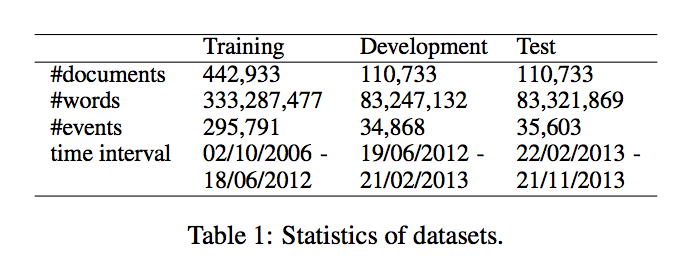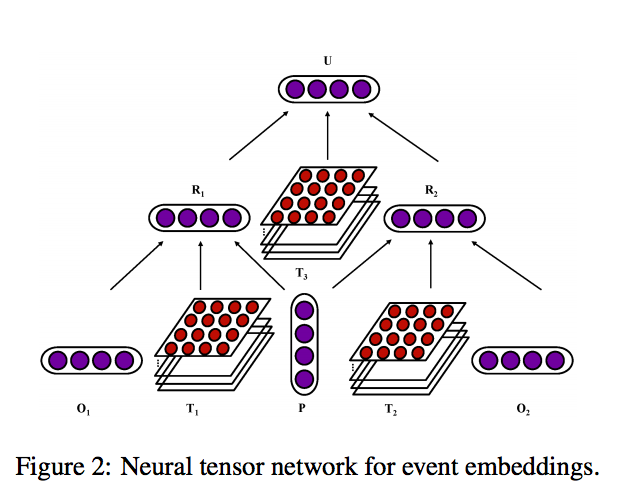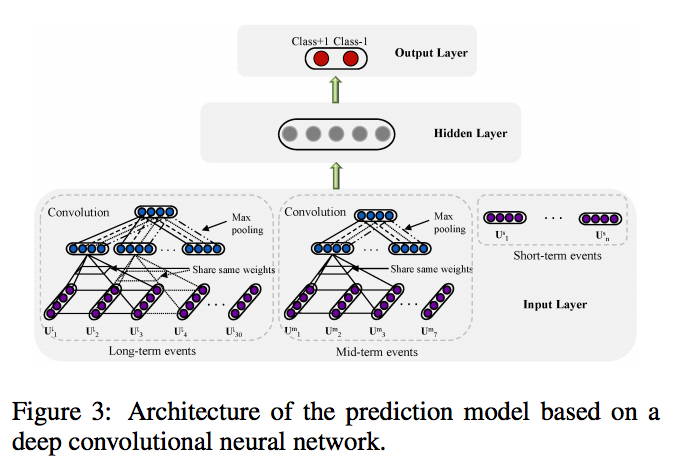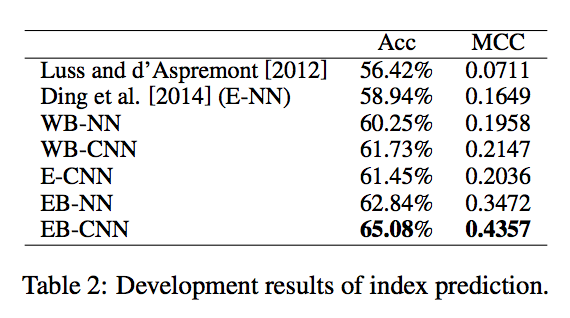# Deep Learning for Event-Driven Stock Prediction (Paper Summary)

Here is the link to the paper.

### Summary

The aim is to be able to predict the price movement using long-term and short-term events, as reported in the news. It is framed as a classification problem. The approach is to first learn event embeddings from the news events. Embeddings for long-term and short-term events are then considered together in a feed-forward network to predict the final class.

### Dataset

They gathered about 10 million news events from Reuters and Bloomberg with timestamps ranging from Oct 2006 to Nov 2013.

Each event has the form $$E = (O_1, P, O_2)$$, where $$P$$ is the action, $$O_1$$ is the actor and $$O_2$$ is the object on which the action is performed. For example, in the event “Google Acquires Smart Thermostat Maker Nest For for \$3.2 billion.”, Actor is Google, Action is acquires and the Object is Nest.### Event EmbeddingsThey convert the news events into event embeddings. They do this using a 2-step process: first converting $$O_1$$ and $$P$$ to $$R_1$$ and $$O_2$$ and $$P$$ to $$R_2$$. $$R_1$$ is computed by:

$R_1 = f(O_1^TT_1^{[1:k]}P + W\begin{bmatrix} O_1 \\ P \\ \end{bmatrix} + b)$

In the second step, $$R_1$$ and $$R_2$$ are combined into the event embedding vector.

### Model Description

The news events are grouped into 3 categories:

• long-term events: Events over the past month
• mid-term events: Events over the past week
• short-term events: Events on the past day of the stock price change

In each case, they combine the events within each day by averaging the event embedding vectors. For long-term and mid-term sequences, they apply a convolutional layer of width 3 to extract localized features. Max pooling is applied to get the final dominant feature from each of them. Ultimately, they end up with feature vectors $$V^C = (V^l, V^m, V^s)$$.This feature vector is fed into a feed-forward neural network with one hidden layer and one output layer. This can be represented as: $$y_{cls} = f(net_{cls}) = \sigma(W_3^T \cdot Y)$$ and $$Y = \sigma(W_2^T \cdot V^C)$$.

### Results• WB-NN: word embeddings input and standard neural network prediction model
• WB-CNN: word embeddings input and convolutional neural network prediction model
• E-CNN: structured events tuple input and convolutional neural network prediction model
• EB-NN: event embeddings input and standard neural network prediction model
• EB-CNN: event embeddings input and convolutional neural network prediction model